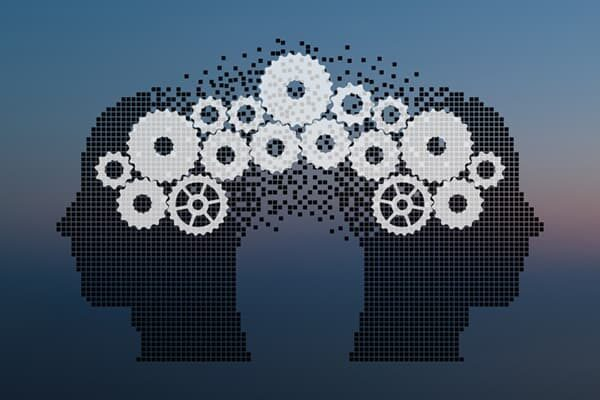R is ubiquitous in the machine learning community. Its ecosystem of more than 8,000 packages makes it the Swiss Army knife of modeling applications.

Similarly, Apache Spark has rapidly become the big data platform of choice for data scientists. Its ability to perform calculations relatively quickly (due to features like in-memory caching) makes it ideal for interactive tasks—such as exploratory data analysis.

Spark provides APIs for four programming languages:

• Scala
• Java
• Python
• R

R (SparkR) is the latest addition and support for it certainly lags the other three languages. In Spark 1.x there was no support for accessing the Spark ML (machine learning) libraries from R. The performance of R code on Spark was also considerably worse than could be achieved using, say, Scala.
These were major barriers to the use of SparkR in modern data science work.

However, Spark 2.x has improved the situation considerably. Crucially, Spark’s new primary data structure (DataSet/DataFrame) is inspired by R’s data frame. This means that all four languages can use this abstraction and obtain performance parity.

In addition, with Spark 2.1, we now have access to much of Spark’s machine learning algorithms from SparkR.

In this article, we’ll see how we can build a random forest classifier in (Spark)R. The code can be run from the industry-standard RStudio or any other R IDE.#### Predicting wine quality with Machine Learning

We’re going to look at using machine learning to predict wine quality based on various characteristics of the wine.

The UCI Machine Learning Repository has a dataset we can use to train our prediction model.

This dataset contains the following features:

• fixed acidity
• volatile acidity
• citric acid
• residual sugar
• chlorides
• free sulfur dioxide
• total sulfur dioxide
• density
• pH
• sulphates
• alcohol
• quality (score between 0 and 10)

There are almost 5000 wines in this dataset, but very few high or low-quality wines. For example, only 5 wines are of the highest quality.

The lack of data at the extremes makes it difficult for the algorithm to learn about these wines, so we’ll follow the approach used in an article by Teja Kodali and classify the wines into

• good (quality is 6–10)
• average (quality is 6)

We chose 6 as “average” as this is the mode of the quality scores—representing over 2000 observations.

#### Data preparation

We’re going to start by obtaining the data and preparing it. To do this we use the readr and dplyr packages.

```library(readr)
library(dplyr)
url <- "https://archive.ics.uci.edu/ml/machine-learning-databases/wine-quality/winequality-white.csv"
df <-
dplyr::mutate(taste = as.factor(ifelse(quality < 6, "bad", ifelse(quality > 6, "good", "average")))) %>%
dplyr::select(-quality)
df <- dplyr::mutate(df, id = as.integer(rownames(df)))```

In this code, we:

• load the data into a data frame
• bin the quality values to create a “taste” categorical feature (as previously discussed)
• add an integer ID column so we can identify the observations more easily

#### Connect to the Spark cluster

For the purposes of this example, we’re connecting to a local Spark cluster. If you have a remote cluster this can be referenced when configuring the session.

When running RStudio (or another IDE) from an application launcher, it may not pick up the SPARK_HOME environment variable (I’m using Linux here), so you need to configure this directly. Alternatively, launch your IDE from a terminal window.

`Sys.setenv(SPARK_HOME="/home/andrew/spark-2.1.0-bin-hadoop2.7")`

We need to load the Spark R package. This is distributed with the Spark installation (in \$SPARK_HOME/R/lib/)_.

`library(SparkR, lib.loc=c(file.path(Sys.getenv("SPARK_HOME"), "R", "lib")))`

Now we can set the Spark session to use our local master node.

`sparkR.session(master="local[*]")`

SparkR doesn’t use normal data frames. It makes use of a distributed data frame that can be spread across the nodes of the cluster. This would normally be loaded from distributed storage such as HDFS or Amazon S3. However, we’ll just convert our small wine data frame to a distributed data frame.

`ddf <- createDataFrame(df)`

Let’s split this into training (70%) and test (30%) datasets. We set a seed so we can get replicable results.

```seed <- 12345
training_ddf <- sample(ddf, withReplacement=FALSE, fraction=0.7, seed=seed)
test_ddf <- except(ddf, training_ddf)```

Note that Spark has not actually computed these datasets yet. It’s lazily building up an execution plan that will be executed when the data is required.

Now we train a model to predict the “taste”.

`model <- spark.randomForest(training_ddf, taste ~ ., type="classification", seed=seed)`

The cluster will now start to do some work. To examine the model parameters—including definitions of all the trees in the forest—use the summary function.

` summary(model)`

We can use our newly trained model to make some predictions on the test dataset. These can be retrieved as a normal (non-distributed) data frame.

```predictions <- predict(model, test_ddf)
prediction_df <- collect(select(predictions, "id", "prediction"))```

Now let’s join the actual “taste” scores to the predicted scores and see whether our model is accurate.

```actual_vs_predicted <-
dplyr::inner_join(df, prediction_df, "id") %>%
dplyr::select(id, actual = taste, predicted = prediction)

mean(actual_vs_predicted\$actual == actual_vs_predicted\$predicted)

table(actual_vs_predicted\$actual, actual_vs_predicted\$predicted)```

In my run, I achieved an accuracy of around 62% (your numbers may vary slightly due to changes to the libraries).

Looking at the tabulated data, very few bad wines were classified as good (6) and vice versa (8). However, the model has trouble separating the average wines from good or bad ones. This is a challenge with our quality encoding approach as wines with a quality score of 7 or 5 are still average.

If we want to use this model in future, we can save it so we don’t have to retrain it every time.

```model_file_path <- "home/andrew/wine_random_forest_model"
write.ml(model, model_file_path)